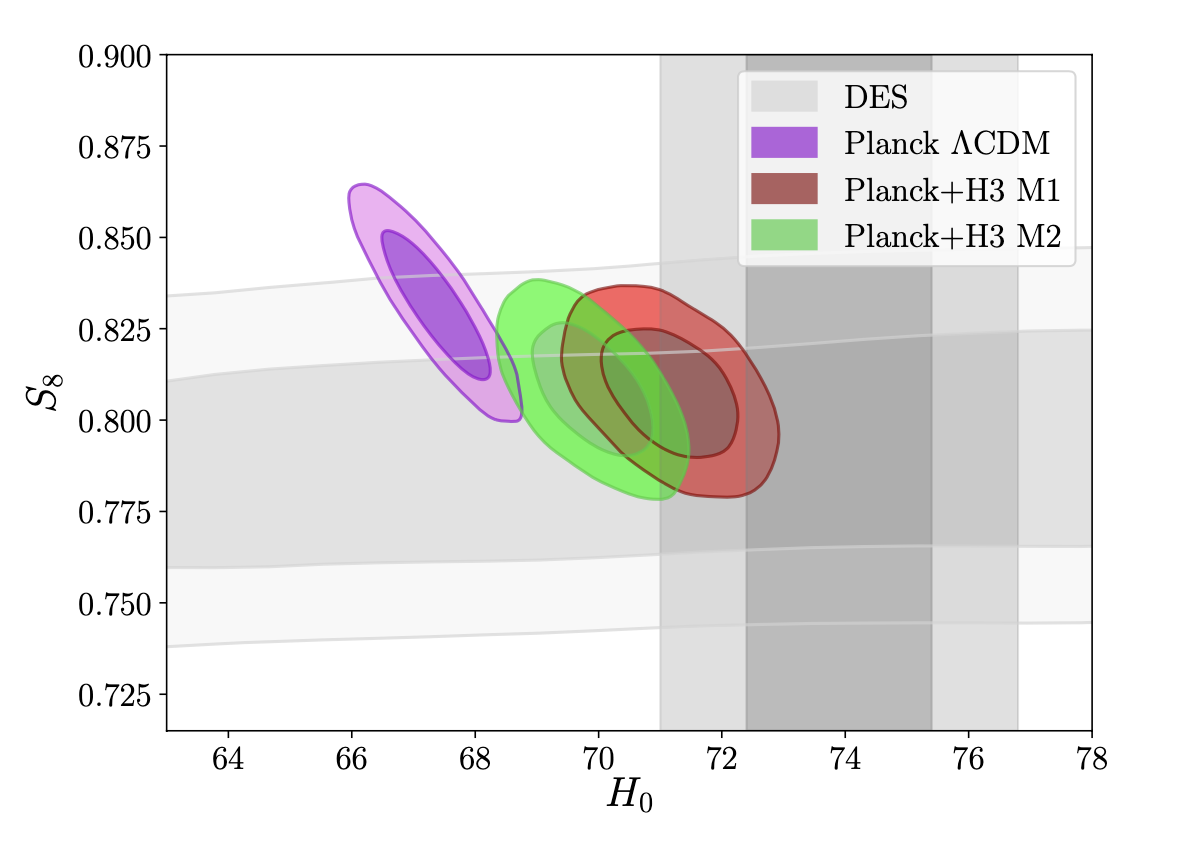# M.A.S.H.: Magnets Add Similarity to Hubbles

Authors: Karsten Jedamzik and Levon Pogosian

First Author’s Institution: Laboratoire de Univers et Particules de Montpellier, Université de Montpellier, 34095 Montpellier, France

Status:  pre-published on arXiv

For the past few years, most astronomers have become closely acquainted with our new favorite bedtime story, The Tale of Two Hubbles. In this tale, our heroes measure two completely different values for the Hubble constant: one in the early universe from the Planck satellite dataset, one in the late universe from Type Ia supernovae. This discrepancy is important, as the Hubble constant is what astronomers use to measure how fast the universe is expanding, and is closely tied to our assumptions about how it all works (see these two bites for more information).

As additional measurements confirm this tension, it could mean our assumptions about the early universe are totally off, and that there is new physics there to be discovered. Alternatively, it might mean that we haven’t accounted for all the important effects that impact these measurements (for instance, voids have been investigated). In this paper, the authors consider how primordial magnetic fields might affect the Planck measurement.Figure 1: The Planck satellite in front of its famous Cosmic Microwave Background image.  Image from the Planck mission website.

### How does Planck measure$H_0$?

Planck data measures the Cosmic Microwave Background (CMB), the oldest light in the Universe we can see. This light was emitted about 300,000 years after the Big Bang (for reference, the Universe is about 13.7 billion years old!), when the Universe cooled enough to allow electrons and protons to bind and form neutral hydrogen atoms— this is called recombination. While a bunch of electrons and protons flying around made the Universe very opaque, effectively trapping the light in a particle soup, a Universe of mostly neutral hydrogen was transparent enough to let the photons (light “particles”) through.

Thus, when cosmologists make predictions about the Universe based on the CMB, we are making predictions about what the universe was like more than 13 billion years ago (for details of how these predictions are made, see this bite). For our purposes, all we need to know is that cosmologists fit a curve to the CMB which depends on six parameters, one of which is$H_0$. This gives us a value for the Hubble constant—$H_0 = 67.8 \pm 0.9 km/s /Mpc$.

### Where do magnetic fields come in?

Since exactly when the CMB is released depends on when recombination happens, the values of the six cosmological parameters we fit also depend on this information. How fast electrons and protons recombine is determined by the ionization rate— the rate at which free particles are being turned into neutral hydrogen— which is proportional to the electron density$n_e$. Basically, the more electrons and protons crowd together, the more likely they are to meet and recombine. Therefore, one way to make the charged particles— which are examples of ordinary matter, or baryons—  recombine faster or slower is to control how close together, or “clumpy” they are.

Putting these terms together, the key parameter to control here is the “clumpiness of baryons,” which is just cosmologist speak for “how much the electrons and protons are grouping together.” The authors do this by turning the dial on Primordial Magnetic Fields (PMF)— weak magnetic fields that could have had an effect on the clumpiness of the soup of electrons and protons. They then calculate the effect these PMFs would have had on modeling the Planck data set and, subsequently, the Hubble constant.

### Did they help?

The authors tried out two clumping models,$M_1$ and$M_2$, based on two different models of the PMFs with associated “clumpiness” parameter b. Both models ramp up the ionization rate, causing recombination to happen sooner. This leaves the shape of the Planck data intact, but the values of the six parameters we like to fit are different, since the Universe was changing rapidly so close to the Big Bang.  The authors show that adding clumpiness from magnetic fields brings the curve of most probable Hubble constant values closer to those derived from late Universe measurements (see Figure 2). Furthermore, the magnetic fields don’t negatively impact the other five parameters that we fit to Planck data, as demonstrated in Figure 3. In fact, they make one of them align slightly better with late Universe measurements!Figure 2: The upper figure shows the$H_0$ probability distribution$P(H_0)$ for a range of$H_0$ values. The purple line shows the original Planck data, and the red and green lines show Planck data with clumping models (named H3 M1 and H3 M2). Note that the clumpy probability distributions overlap with the shaded region on the right, which shows the 68% and 95% confidence level measurements of$H_0$ from the late Universe. The lower figure shows the probability distribution$P(b)$ of the clumping parameter$b$ — the higher its value, the clumpier the electrons and protons. Figure 1 in the paper.Figure 3: The figure shows the$H_0$ contours against that of cosmological parameter which measures matter fluctuations,$S_8$. The purple contour shows the usual fit to the Planck Dataset, while the red and green contours show the values when clumping models H3 M1 and H3 M2 are added. The models with clumping from magnetic fields bring the contours closer to the grey contour, which is a measurement of the parameters from the late Universe. Thus, the authors show that magnetic fields help relieve not only the tension in the Hubble parameter$H_0$, but also make overlap in another cosmological parameter,$S_8$, even better.  Figure 2 in the paper.

### The takeaway

Though these results are promising, chances are we haven’t seen the last of the Tale of Two Hubbles. Whether baryon clumpiness ends up being the smoking gun in the case of the Hubble trouble or not, this paper has demonstrated a purpose: reminding astronomers to account for magnetic fields.